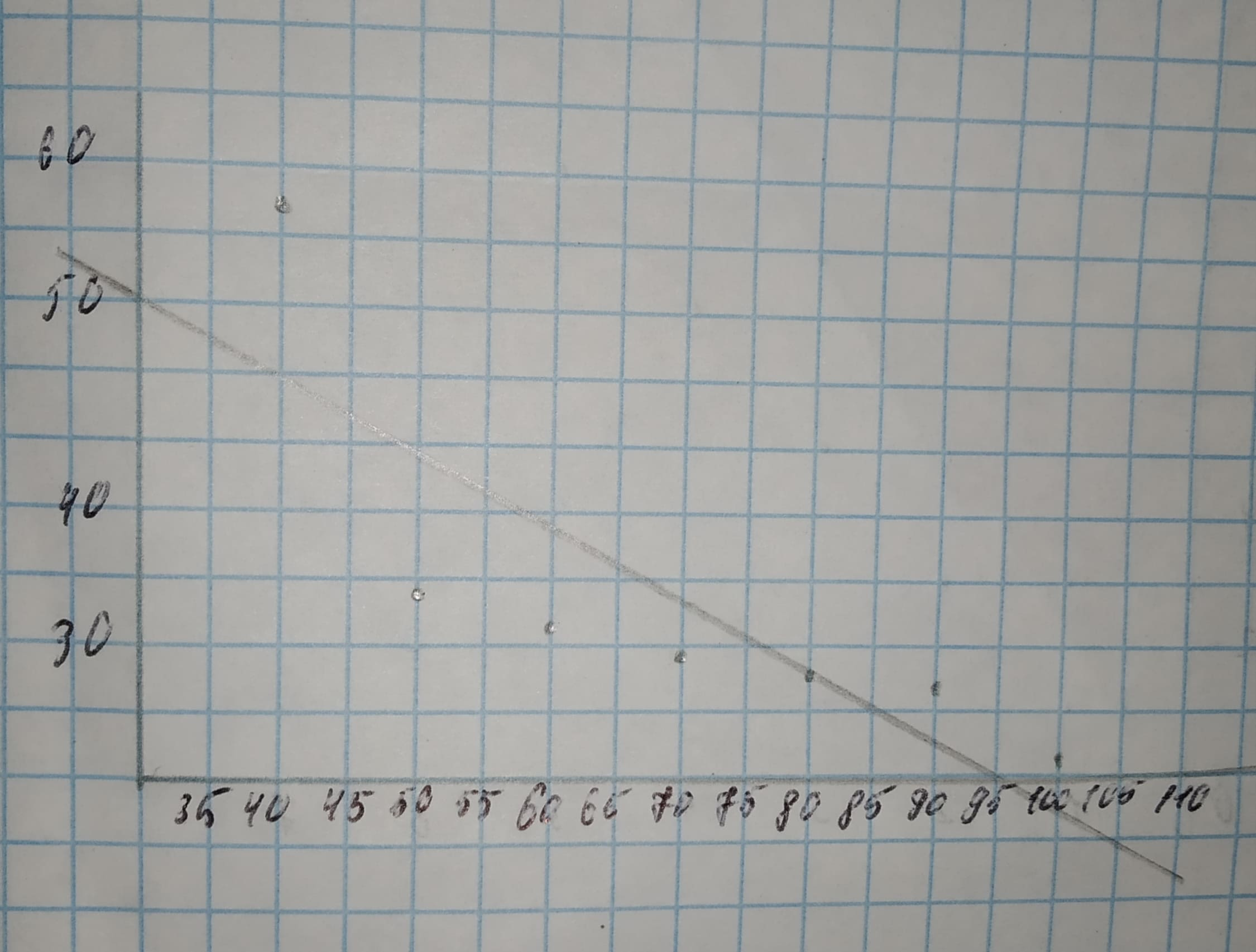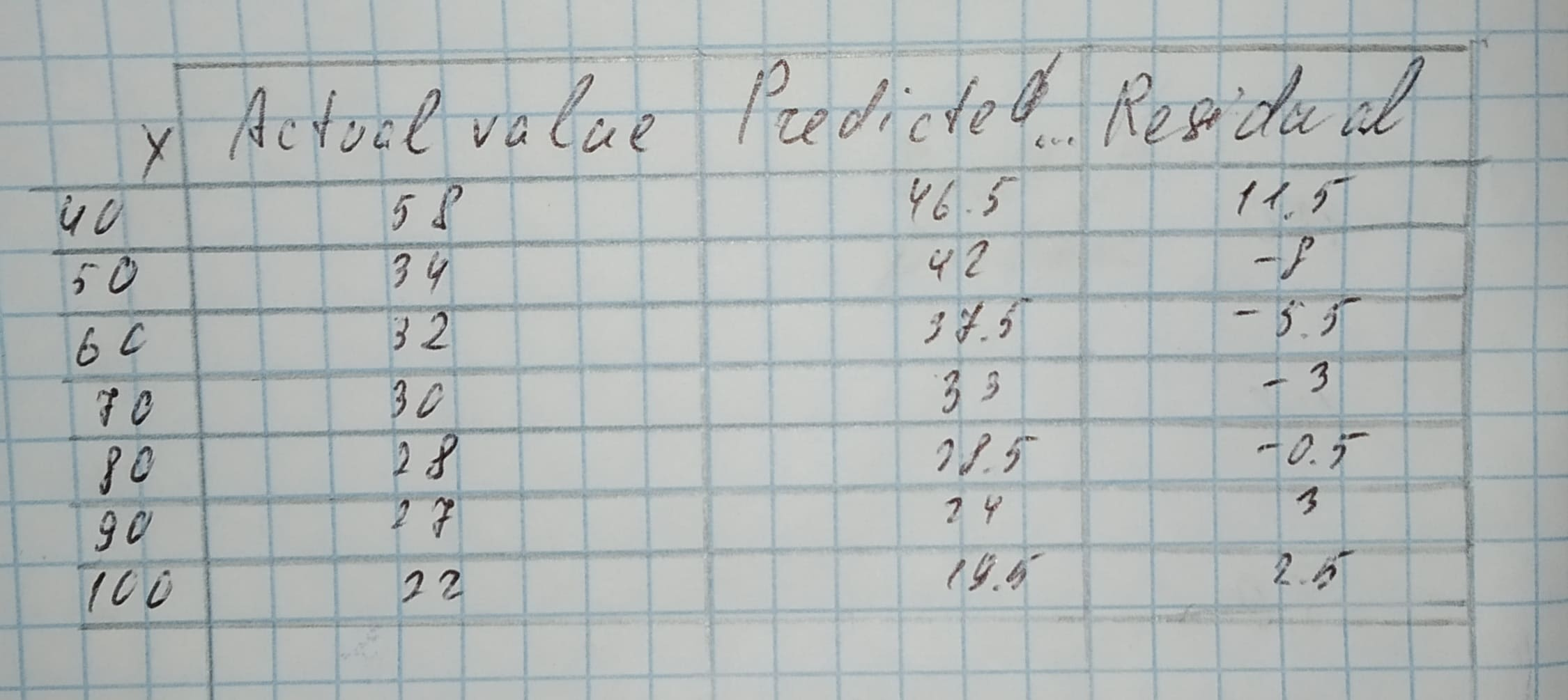# The following data on = soil depth (in centimeters) and y = percentage of montmorillonite in the soil were taken from a scatterplot in the paperBergen 2021-02-03 Answered

The following data on = soil depth (in centimeters) and y = percentage of montmorillonite in the soil were taken from a scatterplot in the paper "Ancient Maya Drained Field Agriculture: Its Possible Application Today in the New River Floodplain, Belize, C.A." (Agricultural Ecosystems and Environment : 67-84):
a. Draw a scatterplot of y versus x.
b. The equation of the least-squares line is 0.45x. Draw this line on your scatterplot. Do there appear to be any large residuals?
c. Compute the residuals, and construct a residual plot. Are there any unusual features in the plot?
$\begin{array}{|cccccccc|}\hline x& 40& 50& 60& 70& 80& 90& 100\\ y& 58& 34& 32& 30& 28& 27& 22\\ \hline\end{array}$
$\left[\stackrel{^}{y}=64.50\right]$.

You can still ask an expert for help

• Questions are typically answered in as fast as 30 minutes

Solve your problem for the price of one coffee

• Math expert for every subject
• Pay only if we can solve itIsma Jimenez

Step 1
a. x is on the horizontal axis and y is on the vertical axis.Step 2
b. Yes. The first point (to the left) lies far away from the line and thus this point will have a large residual.
Step 3
c. The residual is the actual value decreased by the predicted value.Result:
a. Scatterplot
b. Yes
c. Residuals are 11.5, -8, -5.5, -3, -0.5, 3, 2.5